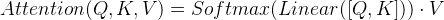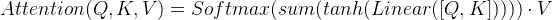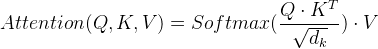# 什么是注意力機制？注意力機制的實現步驟

## 常見的注意力計算規則：

|| ·將Q，K進行縱軸拼接，做一次線性變化，再使用softmax處理獲得結果最后與V做張量乘法。|| ·將Q，K進行縱軸拼接，做一次線性變化后再使用tanh函數激活，然后再進行內部求和，最后使用softmax處理獲得結果再與V做張量乘法.|| ·將Q與K的轉置做點積運算，然后除以一個縮放系數再使用softmax處理獲得結果最后與V做張量乘法。### bmm運算演示:

```# 如果參數1形狀是(b × n × m), 參數2形狀是(b × m × p), 則輸出為(b × n × p)
>>> input = torch.randn(10, 3, 4)
>>> mat2 = torch.randn(10, 4, 5)
>>> res = torch.bmm(input, mat2)
>>> res.size()
torch.Size([10, 3, 5])```

## 注意力機制實現步驟：

```import torch
import torch.nn as nn
import torch.nn.functional as F

class Attn(nn.Module):
def __init__(self, query_size, key_size, value_size1, value_size2, output_size):
"""初始化函數中的參數有5個, query_size代表query的最后一維大小
key_size代表key的最后一維大小, value_size1代表value的導數第二維大小,
value = (1, value_size1, value_size2)
value_size2代表value的倒數第一維大小, output_size輸出的最后一維大小"""
super(Attn, self).__init__()
# 將以下參數傳入類中
self.query_size = query_size
self.key_size = key_size
self.value_size1 = value_size1
self.value_size2 = value_size2
self.output_size = output_size

# 初始化注意力機制實現第一步中需要的線性層.
self.attn = nn.Linear(self.query_size + self.key_size, value_size1)

# 初始化注意力機制實現第三步中需要的線性層.
self.attn_combine = nn.Linear(self.query_size + value_size2, output_size)

def forward(self, Q, K, V):
"""forward函數的輸入參數有三個, 分別是Q, K, V, 根據模型訓練常識, 輸入給Attion機制的
張量一般情況都是三維張量, 因此這里也假設Q, K, V都是三維張量"""

# 第一步, 按照計算規則進行計算,
# 我們采用常見的第一種計算規則
# 將Q，K進行縱軸拼接, 做一次線性變化, 最后使用softmax處理獲得結果
attn_weights = F.softmax(
self.attn(torch.cat((Q, K), 1)), dim=1)

# 然后進行第一步的后半部分, 將得到的權重矩陣與V做矩陣乘法計算,
# 當二者都是三維張量且第一維代表為batch條數時, 則做bmm運算
attn_applied = torch.bmm(attn_weights.unsqueeze(0), V)

# 之后進行第二步, 通過取是用來降維, 根據第一步采用的計算方法,
# 需要將Q與第一步的計算結果再進行拼接
output = torch.cat((Q, attn_applied), 1)

# 最后是第三步, 使用線性層作用在第三步的結果上做一個線性變換并擴展維度，得到輸出
# 因為要保證輸出也是三維張量, 因此使用unsqueeze(0)擴展維度
output = self.attn_combine(output).unsqueeze(0)
return output, attn_weights```

```query_size = 32
key_size = 32
value_size1 = 32
value_size2 = 64
output_size = 64
attn = Attn(query_size, key_size, value_size1, value_size2, output_size)
Q = torch.randn(1,1,32)
K = torch.randn(1,1,32)
V = torch.randn(1,32,64)
out = attn(Q, K ,V)
print(out)
print(out)```

```tensor([[[ 0.4477, -0.0500, -0.2277, -0.3168, -8.4096, -0.5982, 0.1548,
-8.8771, -8.0951. 8.1833. 8.3128. 8.1260, 8.4420. 8.8495.
-0.7774, -0.0995, 0.2629, 0.4957, 1.0922, 0.1428, 0.3024.
-0.2646, -0.0265, 0.0632, 0.3951, 0.1583, 0.1130, 0.5500,
-0.1887, -0.2816, -0.3800, -0.5741, 0.1342, 0.0244, -0.2217,
0.1544, 0.1865, -0.2019, 0.4090, -0.4762, 0.3677, -0.2553,
-0.5199, 0.2290, -0.4407, 0.0663, -8.0182, -8.2168, 0.0913,
-0.2340, 0.1924, -0.3687, 0.1508, 0.3618, -0.0113, 0.2864.
-0.1929, -0.6821, 0.0951, 0.1335, 0.3560, -0.3215
,0.6461,
tensor([[0.0395, 0.0342, 0.0200, 0.0471, 0.0177, 0.0209, 0.0244, 0.0465, 0.0346,
0.0378, 0.0282, 0.0214, 0.0135, 0.0419, 0.0926, 0.0123, 0.0177, 0.0187,
0.0166, 0.8225, 0.0234, 0.0284, 0.0151, 0.0239, 0.0132, 0.0439, 0.0507,
0.0419, 8.0352, 8.0392, 8.0546, 0.0224]], grad_fn=<SoftmaxBackward>)```

Harris角點檢測原理【OpenCV教程】